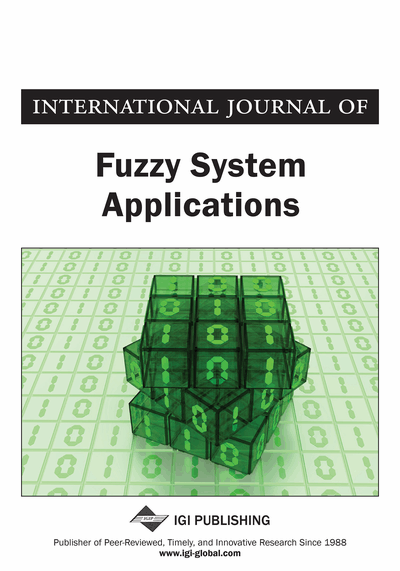# A Fuzzy Multiple Regression Approach for Optimizing Multiple Responses in the Taguchi Method

Abbas Al-Refaie (University of Jordan, Jordan), Ibrahim Rawabdeh (University of Jordan, Jordan), Reema Abu-alhaj (University of Jordan, Jordan), and Issam S. Jalham (University of Jordan, Jordan)
DOI: 10.4018/ijfsa.2012070102
Available
\$29.50
No Current Special Offers

## Abstract

The fuzzy regression has been found effective in modeling the relationship between the dependent variable and independent variables when a high degree of fuzziness is involved and only a few data sets are available for model building. This research, therefore, proposes an approach for optimizing multiple responses in the Taguchi method using fuzzy regression and desirability function. The statistical regression is formulated for the signal to noise (S/N) ratios of each response replicate. Then, the optimal factor levels for each replicate are utilized in building fuzzy regression model. The desirability function, pay-off matrix, and the deviation function are finally used for formulating the optimization models for the lower, mean, and upper limits. Two case studies investigated in previous literature are employed for illustration; where in both case studies the proposed approach efficiently optimized processes performance.
Article Preview
Top

## Introduction

The introduction of the robust design by Taguchi (1991), or the so-called Taguchi method, has become a powerful tool for producing high-quality products at low cost. In this method, orthogonal arrays (OAs), are usually utilized to reduce the number of experiments under permissive reliability. The signal-to-noise (S/N) ratio is then employed to decide the optimal factor levels. The Taguchi method has been widely accepted for robust design in many business applications to optimize a single quality response of a process or product (Yang, 2001; Ghani et al., 2004; Chih et al., 2005, Shing & Hwee, 2005; Shu et al., 2006; Barend & Villiers, 2007; Li et al., 2008). Recently, the optimization of multiple responses in the Taguchi method has received significant research attention. Several techniques are adopted for this purpose, including a trade-off between quality loss and productivity (Phadke, 1989), multiple response S/N ratio (Antony, 2001; Lin, 2002; Al-Refaie et al., 2010), data envelopment analysis (Al-Refaie et al., 2009), grey relational analysis and desirability function approach (Lin, 2004; Palanikumar et al., 2006; Pan et al., 2007; Al-Refaie et al., 2008; Lin & Lee, 2009; Chen et al., 2010), principal components analysis (Tong et al., 2005; Fung & Kang, 2005; Huang & Lin, 2008; Gauri & Chakraborty, 2009), fuzzy logic approach (Tarng et al., 2000; Lin & Lin, 2005; Wang & Jean, 2006; Chang & Lu, 2007), and multiple regression-based integration approach (Gopalsamy et al., 2009; Pal & Gauri, 2010). Although statistical regression has many applications (Shu et al., 2006; Gopalsamy et al., 2009), problems can still occur in the following situations, including number of observations is inadequate (small data set), difficulties verifying distribution assumptions, vagueness in the relationship between input and output variables, ambiguity of events or degree to which they occur, and inaccuracy and distortion introduced by linearization.

The fuzzy regression analysis uses fuzzy numbers, which can be expressed as intervals with membership values as the regression coefficients, which was first introduced by Tanaka et al. (1982). The fuzzy regression (Toly, 2011a, 2011b; Al-Refaie & Li, 2011) has been found to be more appealing than statistical regression in estimating the relationship between the dependent variable and independent variables when a high degree of fuzziness is involved and only a few data sets are available for model building. In a fuzzy regression model, the deviations between the observed values and the estimated values are expressed as the possibilities that the system has. The relationship between dependent and independent variables is defined by using fuzzy concept rather than statistical concept. In other words, a fuzzy regression model aims to build a model which contains all observed data in the estimated fuzzy numbers.

## Complete Article List

Search this Journal:
Reset
Volume 12: 1 Issue (2023)
Volume 11: 4 Issues (2022)
Volume 10: 4 Issues (2021)
Volume 9: 4 Issues (2020)
Volume 8: 4 Issues (2019)
Volume 7: 4 Issues (2018)
Volume 6: 4 Issues (2017)
Volume 5: 4 Issues (2016)
Volume 4: 4 Issues (2015)
Volume 3: 4 Issues (2013)
Volume 2: 4 Issues (2012)
Volume 1: 4 Issues (2011)
View Complete Journal Contents Listing